## Polynomials Involving Perimeter, Area, and Volume

### Learning Outcomes

• Write polynomials involving perimeter, area, and volume

In this section we will explore ways that polynomials are used in applications of perimeter, area, and volume. First, we will see how a polynomial can be used to describe the perimeter of a rectangle.

### Example

A rectangular garden has one side with a length of $x+7$ and another with a length $2x + 3$. Find the perimeter of the garden.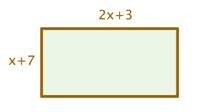### Try It

In the following video you are shown how to find the perimeter of a triangle whose sides are defined as polynomials.

The area of a circle can be found using the radius of the circle and the constant pi in the formula $A=\pi{r^2}$. In the next example we will use this formula to find a polynomial that describes the area of an irregular shape.

### Example

Find a polynomial for the area of the shaded region of the figure.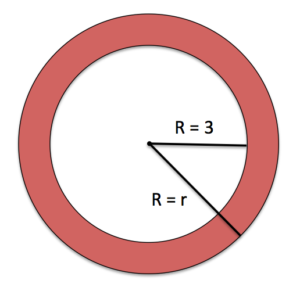In the video that follows, you will be shown an example of determining the area of a rectangle whose sides are defined as polynomials.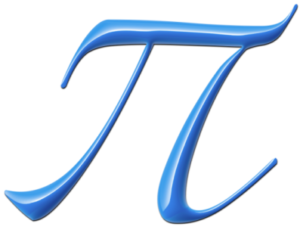Pi

It is easy to confuse pi as a variable because we use a greek letter to represent it.  We use a greek letter instead of a number because nobody has been able to find an end to the number of digits of pi.  To be precise and thorough, we use the greek letter as a way to say: “we are including all the digits of pi without having to write them”. The expression for the area of the shaded region in the example above included both the variable r, which represented an unknown radius and the number pi.  If we needed to use this expression to build a physical object or instruct a machine to cut specific dimensions, we would round pi to an appropriate number of decimal places.

In the next example, we will write the area for a rectangle in two different ways, one as the product of two binomials and the other as the sum of four rectangles. Because we are describing the same shape two different ways, we should end up with the same expression no matter what way we define the area.

### Example

Write two different polynomials that describe the area of of the figure. For one expression, think of the rectangle as one large figure, and for the other expression, think of the rectangle as the sum of $4$ different rectangles.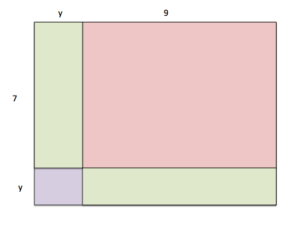The last example we will provide in this section is one for volume.  The volume of regular solids such as spheres, cylinders, cones and rectangular prisms are known.  We will find an expression for the volume of a cylinder, which is defined as $V=\pi{r^2}h$.

### Example

Define a polynomial that describes the volume of the cylinder shown in the figure: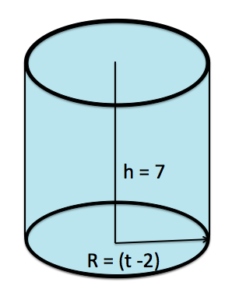In this last video, we present another example of finding the volume of a cylinder whose dimensions include polynomials.

In this section we defined polynomials that represent perimeter, area and volume of well-known shapes.  We also introduced some convention about how to use and write $\pi$ when it is combined with other constants and variables. The next application will introduce you to cost and revenue polynomials.  Next we will see that cost and revenue equations can be polynomials.

## Contribute!

Did you have an idea for improving this content? We’d love your input.